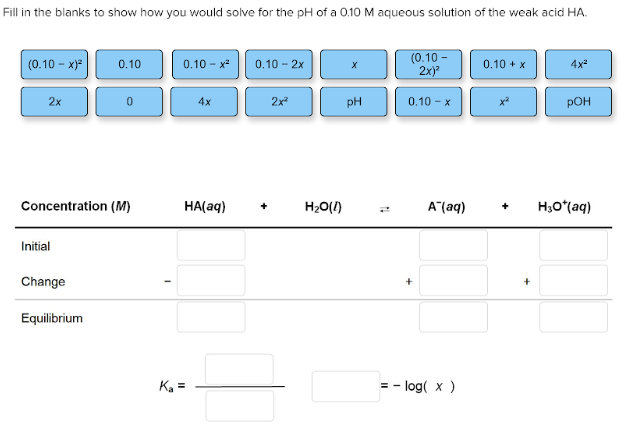# Fill in the blanks to show how you would solve for the pH of a 0.10 M aqueous solution of the weak acid HA. (0.10-x)^2, 0.10, 0.10-x^2, 0.10-2x, x, (0.10-2x)^2, 0.10+x, 4x^2, 2x, 0, 4x, 2x^2, pH, 0.10-x, x^2, pOH Concentration (M) HA(aq) + H2O(l) <-> A-(aq) + H3O+(aq) Ka = [__]/[__] -log(x) = [__]